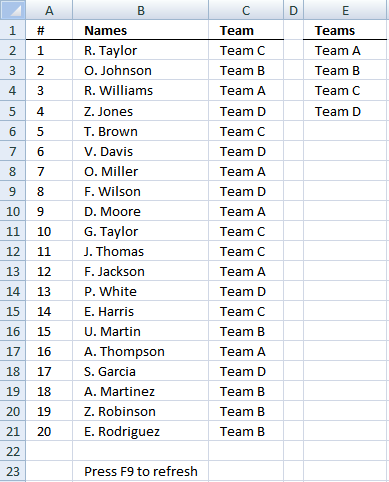Author: Oscar Cronquist Article last updated on January 02, 2019This blog article describes how to create teams randomly. There are twenty names in column B and four teams in column E. There will be five team members in each team. In column C a team is randomly selected in each cell.

Array formula in cell C2:

=INDEX(\$E\$2:\$E\$5, LARGE((COUNTIF(\$C\$1:C1, \$E\$2:\$E\$5)<5)*(ROW(\$1:\$4)), RANDBETWEEN(1, SUMPRODUCT(--(COUNTIF(\$C\$1:C1, \$E\$2:\$E\$5)<5)))))

### How to create an array formula

1. Copy the aray formula above (Ctrl + c)
2. Double click cell B2
3. Paste (Ctrl + v)
4. Press and hold Ctrl + Shift simultaneously
5. Press Enter
6. Release all keys

If you made the above steps correctly the formula now has a beginning and ending curly bracket, like this:
{=array_formula}

Don't enter these characters yourself, they appear automatically.

### Customize formula

COUNTIF(\$C\$1:C1, \$E\$2:\$E\$5)<5 makes sure that each team has max five team members.

ROW(\$1:\$4) gives a row number to each team. Example, if you have three teams, change it to ROW(\$1:\$3) or the formula won't work.

### Explaining formula in cell C2

#### Step 1 - Make sure that not more than 5 names have been assigned the same team

The COUNTIF function counts values based on a condition or criteria, the first argument contains an expanding cell reference, it grows when the cell is copied to cells below. This makes the formula aware of values displayed in cells above.

COUNTIF(\$C\$1:C1, \$E\$2:\$E\$5)<5

becomes

COUNTIF("Team",{"Team A"; "Team B"; "Team C"; "Team D"})<5

becomes

{0; 0; 0; 0}<5

and returns {TRUE; TRUE; TRUE; TRUE}.

#### Step 2 - Multiply with array containing row numbers

(COUNTIF(\$C\$1:C1, \$E\$2:\$E\$5)<5)*(ROW(\$1:\$4))

becomes

{TRUE; TRUE; TRUE; TRUE}*(ROW(\$1:\$4)

becomes

{TRUE; TRUE; TRUE; TRUE}*{1;2;3;4}

and returns

{1;2;3;4}

#### Step 3 - Extract random row number

The LARGE function returns the k-th largest number, k is calculated based on how many teams that have been shown up until this cell.

LARGE((COUNTIF(\$C\$1:C1, \$E\$2:\$E\$5)<5)*(ROW(\$1:\$4)), RANDBETWEEN(1, SUMPRODUCT(--(COUNTIF(\$C\$1:C1, \$E\$2:\$E\$5)<5))))

becomes

LARGE({1;2;3;4}, RANDBETWEEN(1, SUMPRODUCT(--(COUNTIF(\$C\$1:C1, \$E\$2:\$E\$5)<5))))

SUMPRODUCT function returns the number of team names that have not yet been fully assigned to players.

LARGE({1;2;3;4}, RANDBETWEEN(1, SUMPRODUCT(--({TRUE; TRUE; TRUE; TRUE}))))

becomes

LARGE({1;2;3;4}, RANDBETWEEN(1, SUMPRODUCT({1; 1; 1; 1})))

becomes

LARGE({1;2;3;4}, RANDBETWEEN(1, 4))

becomes

LARGE({1;2;3;4}, 3).

and returns 3. This is a random number.

#### Step 4 - Return value

The INDEX function returns a value based on a cell reference and column/row numbers.

INDEX(\$E\$2:\$E\$5, LARGE((COUNTIF(\$C\$1:C1, \$E\$2:\$E\$5)<5)*(ROW(\$1:\$4)), RANDBETWEEN(1, SUMPRODUCT(--(COUNTIF(\$C\$1:C1, \$E\$2:\$E\$5)<5)))))

becomes

INDEX(\$E\$2:\$E\$5, 3)

and returns "Team C" in cell C2.